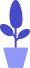# A Preliminary Study of Python Exception

Estimated reading: 2 minutes 56 views

Syntax errors are often encountered when writing Python programs. For example, as you learned earlier, if a variable name starts with a number, Python will immediately give an error in red. If we forget to indent somewhere, Python will also give an error message telling us that an indent is needed. If there is no colon after the if statement when using the if statement, there `:`will also be an error message.

Generally speaking, syntax errors are relatively simple, and when you see the prompts given by Python, it is clear where the error occurred. Common syntax errors include spelling errors, indentation errors, and programs that do not conform to Python’s syntax specifications. In addition to syntax errors, in the process of software development, there will be another kind of errors, called exceptions .

Exceptions are errors thrown by a program while it is running. Once an exception occurs, the Python interpreter will terminate the program and output a red warning message. Let’s first look at an exception example, the code is as follows.

``````numberEight=8
stringEight="8"
print(numberEight+stringEight)``````

We define two variables, one is numberEight, which represents the number 8, and the other is stringEight, which represents the character “8”. Now, we connect these two variables with the operator “+” sign, what will happen?

Let’s run this code and get an error message, as shown in Figure 1.

Figure 1
You can see that Python gives an exception message, it will indicate that the code that caused the exception is on line 3, the error statement is print (numberEight+stringEight), and it is clearly a type error (TypeError), that is, the operator symbol “+” does not Operations of int type and str type are supported.

Let’s look at another example, this time use the variable numberEight to remove 0 to see what kind of error you get. The code is as follows.

``````numberEight=8
print(numberEight/0)``````

Run the code and get the error message shown in Figure 2.